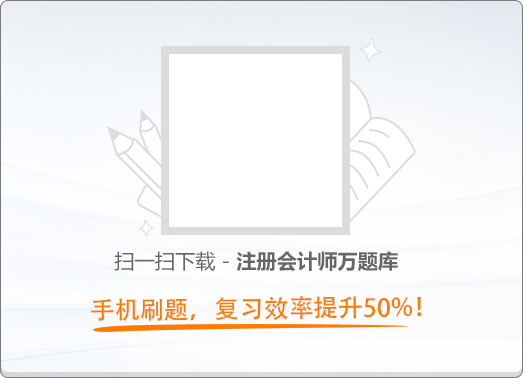150:002017年注册会计师《公司战略与风险管理》真题

1
(单项选择题)

• A.

家电业务的多数产品进入成熟期，公司在家电行业竞争优势显著。公司应当对该业务加大投资力度，以维持公司在行业中的优势地位

• B.

新能源行业发展潜力巨大、前景广阔，公司在该领域竞争优势不足。公司应当对新能源业务进行重点投资，以提高市场占有率

• C.

房地产业进入“寒冬”期，公司的房地产业务始终没有获利。公司应当果断地从该业务中撤出

• D.

生物制药行业近年来发展迅猛，公司收购的一家生物制药企业由弱到强，竞争优势日益显现。公司应当在短期内优先供给其所需资源，支持该业务继续发展

• A
• B
• C
• D

2
(单项选择题)

• A.

导入期

• B.

成长期

• C.

成熟期

• D.

衰退期

• A
• B
• C
• D

3
(单项选择题)

2010年，R国汽车制造商G公司预计随着绿色环保理念的普及和政府相关产业政策的推出，R国的新能源汽车产业将迎来一个巨大的发展机遇；其本国竞争对手车制造商S公司，将凭借雄厚的资金实力和强大的科研能力，把投资和研发的重点转向新能源汽车领域。G公司对S公司的上述分析属于（　）。

• A.

财务能力分析

• B.

成长能力分析

• C.

适应变化能力分析

• D.

快速反应能力分析

• A
• B
• C
• D

4
(单项选择题)

• A.

增长型战略

• B.

扭转型战略

• C.

多种经营战略

• D.

防御型战略

• A
• B
• C
• D

5
(单项选择题)

• A.

缺乏明确的发展战略

• B.

发展战略实施不到位

• C.

发展战略过于激进，脱离企业实际能力或偏离主业

• D.

发展战略因主观原因频繁变动

• A
• B
• C
• D

6
(单项选择题)

• A.

控制环境

• B.

风险评估

• C.

控制活动

• D.

监控

• A
• B
• C
• D

7
(单项选择题)

• A.

职能结构

• B.

事业部结构

• C.

战略业务单位结构

• D.

矩阵制组织结构

• A
• B
• C
• D

8
(单项选择题)

P公司是一家生产经营日化用品的跨国公司，其母公司设立在U国，在其他国家设立了20余个子公司。在该公司的经营过程中，母公司将产品的研发技术和新产品提供给各个子公司，子公司也会把在当地畅销的产品提供给母公司和其他子公司。P公司国际化经营的战略类型属于（　）。

• A.

国际战略

• B.

跨国战略

• C.

全球化战略

• D.

多国本土化战略

• A
• B
• C
• D

9
(单项选择题)

• A.

相互适应，自行调整

• B.

技艺（知识）标准化

• C.

工作过程标准化

• D.

工作成果标准化

• A
• B
• C
• D

10
(单项选择题)

• A.

该方法降低了存货变质、陈旧或过时的可能性

• B.

该方法可能导致生产环节一旦出错则弥补空间小

• C.

该方法能够减少对供应商的依赖

• D.

该方法避免了因需求突然变动而导致大量产品无法出售的情况

• A
• B
• C
• D

11
(单项选择题)

• A.

专业自保

• B.

损失融资

• C.

风险资本

• D.

保险

• A
• B
• C
• D

12
(单项选择题)

• A.

正推法

• B.

后推法

• C.

前推法

• D.

逆推法

• A
• B
• C
• D

13
(单项选择题)

2007～2013年，S公司在作为P公司最大的元器件和闪存供应商的同时，推出了系列智能手机和平板电脑，成为P公司在智能手机和平板电脑市场主要的竞争对手。P公司很想摆脱对S公司的依赖，但由于S公司在生产关键零部件方面的能力显著强于其他公司，因而在短期内P公司仍然离不开S公司。这一案例中，影响S公司对P公司讨价还价能力的主要因素是（　）。

• A.

业务量

• B.

产品差异化程度与资产专用性程度

• C.

纵向一体化程度

• D.

信息掌握程度

• A
• B
• C
• D

14
(单项选择题)

M世纪90年代，光美公司在国内推出微波炉产品。目前光美公司已建成覆盖全国的营销网络，包括电商销售平台、数以千计的超市专卖柜和实体店以及十几个仓储物流中心。近年来不少企业试图进入微波炉行业，均未能成功。光美公司给潜在进入者设置的进入障碍是（　）。

• A.

现有企业对关键资源的控制

• B.

行为性障碍

• C.

规模经济

• D.

现有企业的市场优势

• A
• B
• C
• D

15
(单项选择题)

• A.

前向一体化战略

• B.

后向一体化战略

• C.

多元化战略

• D.

密集型战略

• A
• B
• C
• D

16
(单项选择题)

• A.

聘请咨询公司实施广告策略

• B.

物流配送产品

• C.

通过互联网进行广告宣传

• D.

生产设备的维修

• A
• B
• C
• D

17
(单项选择题)

• A.

专题讨论

• B.

实地查验

• C.

穿行测试

• D.

审阅与检查

• A
• B
• C
• D

18
(单项选择题)

• A.

流程图分析法

• B.

事件树分析法

• C.

决策树分析法

• D.

敏感性分析法

• A
• B
• C
• D

19
(单项选择题)

• A.

战略风险

• B.

市场风险

• C.

产业风险

• D.

技术风险

• A
• B
• C
• D

20
(单项选择题)

• A.

“防御者”战略

• B.

“抗衡者”战略

• C.

“扩张者”战略

• D.

“躲闪者”战略.

• A
• B
• C
• D

21
(单项选择题)

• A.

寻求市场

• B.

寻求效率

• C.

寻求资源

• D.

寻求现成资产

• A
• B
• C
• D

22
(单项选择题)

• A.

多元化战略

• B.

市场开发战略

• C.

市场渗透战略

• D.

产品开发战略

• A
• B
• C
• D

23
(单项选择题)

• A.

集中成本领先战略

• B.

混合战略

• C.

成本领先战略

• D.

失败战略

• A
• B
• C
• D

24
(单项选择题)

• A.

情景分析法

• B.

敏感性分析法

• C.

马尔科夫分析法

• D.

风险评估分析法

• A
• B
• C
• D